# GSEB Solutions Class 7 Maths Chapter 2 Fractions and Decimals Ex 2.1

Gujarat Board GSEB Textbook Solutions Class 7 Maths Chapter 2 Fractions and Decimals Ex 2.1 Textbook Questions and Answers.

## Gujarat Board Textbook Solutions Class 7 Maths Chapter 2 Fractions and Decimals Ex 2.1Question 1.
Solve:
(i) 2 – $$\frac { 3 }{ 5 }$$
(ii) 4 + $$\frac { 7 }{ 8 }$$
(iii) $$\frac { 3 }{ 5 }$$ + $$\frac { 2 }{ 7 }$$
(iv) $$\frac { 9 }{ 11 }$$ – $$\frac { 4 }{ 15 }$$
(v) $$\frac { 7 }{ 10 }$$ + $$\frac { 2 }{ 5 }$$ + $$\frac { 3 }{ 2 }$$
(vi) 2$$\frac { 2 }{ 3 }$$ + 3$$\frac { 1 }{ 2 }$$
(vii) 8$$\frac { 1 }{ 2 }$$ – 3$$\frac { 5 }{ 8 }$$
Solution:
(i) 2 – $$\frac { 3 }{ 5 }$$ = $$\frac { 2 }{ 1 }$$ – $$\frac { 3 }{ 5 }$$
= $$\frac { 2(5) – 1(3) }{ 5 }$$
= $$\frac { 10 – 3 }{ 5 }$$
= $$\frac { 7 }{ 5 }$$

(ii) 4 + $$\frac { 7 }{ 8 }$$
= $$\frac { 4(8) + 1(7) }{ 8 }$$
= $$\frac { 32 + 7 }{ 8 }$$
= $$\frac { 39 }{ 8 }$$ or 4$$\frac { 7 }{ 8 }$$

(iii) $$\frac { 3 }{ 5 }$$ + $$\frac { 2 }{ 7 }$$
= $$\frac { 7(3) + 5(2) }{ 5 }$$
= $$\frac { 21 + 10 }{ 35 }$$
= $$\frac { 31 }{ 35 }$$

(iv) $$\frac { 9 }{ 11 }$$ – $$\frac { 4 }{ 15 }$$
= $$\frac { 135 – 44}{ 165 }$$
= $$\frac { 91 }{ 165 }$$

(v) $$\frac { 7 }{ 10 }$$ + $$\frac { 2 }{ 5 }$$ + $$\frac { 3 }{ 2 }$$
= $$\frac { 1(7) + 2(2)+5(3) }{ 10 }$$    [∵ LCM of 2, 10 and 5 is 10]
= $$\frac { 7 + 4+15 }{ 10 }$$

(vi) 2$$\frac { 2 }{ 3 }$$ + 3$$\frac { 1 }{ 2 }$$
= $$\frac { 8 }{ 3 }$$ + $$\frac { 7 }{ 2 }$$
= $$\frac { 2(8) + 3(7) }{ 6 }$$
= $$\frac { 16 + 21) }{ 6 }$$              [∵ LCM of 3 and 2 is 6 ]
= $$\frac { 37 }{ 6 }$$ (= 6$$\frac { 1 }{ 6 }$$ )

(vii) 8$$\frac { 1 }{ 2 }$$ – 3$$\frac { 5 }{ 8 }$$
= $$\frac { 17 }{ 2 }$$ – $$\frac { 29 }{ 2 }$$
= $$\frac { 4(17) – 1(29) }{ 8 }$$         [∵ LCM of 3 and 2 is 6 ]
= $$\frac { 68-29 }{ 8 }$$
= $$\frac { 39 }{ 8 }$$(= 4$$\frac { 7 }{ 8 }$$ )Question 2.
Arrange the following in descending order:
(i) $$\frac { 2 }{ 9 }$$, $$\frac { 2 }{ 3 }$$, $$\frac { 8 }{ 21 }$$
(ii) $$\frac { 1 }{ 5 }$$, $$\frac { 3 }{ 7 }$$, $$\frac { 7 }{ 10 }$$
Solution:
(i) $$\frac { 2 }{ 9 }$$, $$\frac { 2 }{ 3 }$$, $$\frac { 8 }{ 21 }$$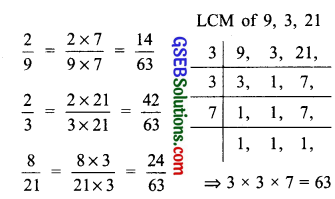Since, 42 > 24 > 14
∴ $$\frac { 42 }{ 63 }$$ > $$\frac { 24 }{ 63 }$$ > $$\frac { 14 }{ 63 }$$
Thus $$\frac { 2 }{ 3 }$$, $$\frac { 8 }{ 21 }$$, $$\frac { 2 }{ 9 }$$ are in descending order.

(ii) $$\frac { 1 }{ 5 }$$, $$\frac { 3 }{ 7 }$$, $$\frac { 7 }{ 10 }$$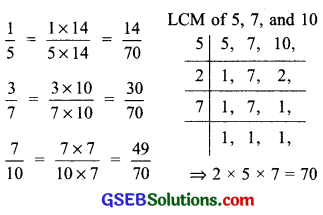Since, 49 > 30 > 14
∴ $$\frac { 49 }{ 70 }$$ > $$\frac { 30 }{ 70 }$$ > $$\frac { 14 }{ 70 }$$
Thus $$\frac { 7 }{ 10 }$$, $$\frac { 3 }{ 7 }$$, $$\frac { 1 }{ 5 }$$ are in descending order.

Question 3.
In a “magic square”, the sum of the numbers in each row, in each column and along the diagonals is the same. Is this a magic square?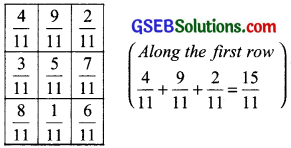Since, the sum of numbers in each case is the same, therefore, it is a magic square.Question 4.
A rectangular sheet of paper is 12$$\frac { 1 }{ 2 }$$ cm long and 10 $$\frac { 2 }{ 3 }$$ cm wide. Find its perimeter.
Solution:
Length = 12$$\frac { 1 }{ 2 }$$cm = $$\frac { 25 }{ 2 }$$cm
Width = 10$$\frac { 2 }{ 3 }$$cm = $$\frac { 32 }{ 3 }$$cm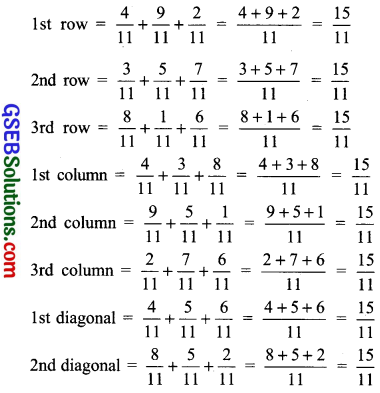Since, the sum of numbers in each case is the same, therefore, it is a magic square.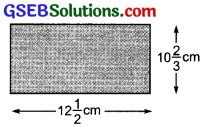∵ Perimeter of a rectangle = 2[Length + Width]
∴ The perimeter of the rectangular piece of paper
= 2[ $$\frac { 25 }{ 2 }$$ + $$\frac { 32 }{ 3 }$$ ]cm
= 2[ $$\frac { 75+64 }{ 6 }$$ ]cm
= 2[ $$\frac { 139 }{ 6 }$$ ]cm         [∵ LCM of 2 and 3 is 6 ]
= $$\frac { 139 }{ 3 }$$cm
= 46 $$\frac { 1 }{ 3 }$$cm

Question 5.
Find the perimeter of (i) ∆ABE and (ii) the rectangle BCDE in this figure. Whose perimeter is greater?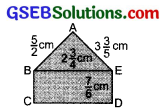Solution:
(i) Perimeter of ∆ABE = AB + BE + AE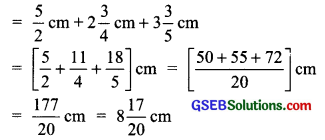(ii) Perimeter of rectangle BCDE = 2 [BE + DE]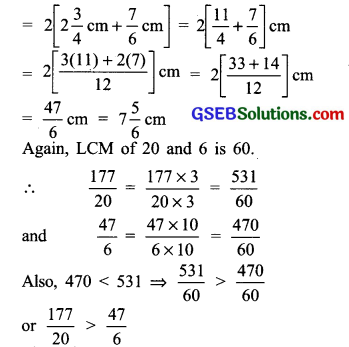Thus, the perimeter of ∆ ABE is greater.Question 6.
Salil wants to put a picture in a frame. The picture is 7$$\frac { 3 }{ 5 }$$cm wide. To fit in the frame the picture cannot be more than 7$$\frac { 3 }{ 10 }$$cm wide. How much should the picture be trimmed?
Solution:
Width of the picture = 7$$\frac { 3 }{ 5 }$$cm = $$\frac { 38 }{ 5 }$$cm
Required width of the picture
= 7$$\frac { 3 }{ 10 }$$cm = $$\frac { 73 }{ 10 }$$cm
∴ The picture is to be trimmed by [ $$\frac { 38 }{ 5 }$$ – $$\frac { 73 }{ 10 }$$ ]cm
∵ LCM of 5 and 10 = 10
∴ [ $$\frac { 38 }{ 5 }$$ – $$\frac { 73 }{ 10 }$$ ]cm
= $$\frac { 76-73 }{ 10 }$$cm
= $$\frac { 3 }{ 10 }$$cm
Thus, the picture should be trimmed by $$\frac { 3 }{ 10 }$$cm.

Question 7.
Ritu ate $$\frac { 3 }{ 5 }$$ part of an apple and the remaining apple was eaten by her brother Somu. How much part of the apple did Somu eat? Who had the larger share? By how much?
Solution:
Ritu ate $$\frac { 3 }{ 5 }$$ part of an apple.
Somu ate the remaining part, i.e. 1 – $$\frac { 3 }{ 5 }$$ = $$\frac { 2 }{ 5 }$$ part
Again 3 > 2
∴ $$\frac { 3 }{ 5 }$$ > $$\frac { 2 }{ 5 }$$ ⇒ Ritu had the larger share.
Again
$$\frac { 3 }{ 5 }$$ – $$\frac { 2 }{ 5 }$$ = $$\frac { 1 }{ 5 }$$
Thus, Ritu had the larger share by $$\frac { 1 }{ 5 }$$ part of an apple.Question 8.
Michael finished colouring a picture in $$\frac { 7 }{ 12 }$$ hour. Vaibhav finished colouring the same picture in $$\frac { 3 }{ 4 }$$ hour. Who worked longer? By what fraction was it longer?
Solution:
Michael takes $$\frac { 7 }{ 12 }$$ hour and Vaibhav takes $$\frac { 3 }{ 4 }$$ hour colouring a picture.
And
$$\frac { 7 }{ 12 }$$ = $$\frac { 7×1 }{ 12×1 }$$ = $$\frac { 7 }{ 12 }$$;
$$\frac { 3 }{ 4 }$$ x $$\frac { 3 }{ 3 }$$ = $$\frac { 9 }{ 12 }$$
Since $$\frac { 9 }{ 12 }$$ > $$\frac { 7 }{ 12 }$$, So Vaibhav worked longer.
Also,
$$\frac { 9 }{ 12 }$$ – $$\frac { 7 }{ 12 }$$ = $$\frac { 2 }{ 12 }$$ or $$\frac { 1 }{ 6 }$$
Thus, Vaibhav worked longer by $$\frac { 1 }{ 6 }$$ of an hour.